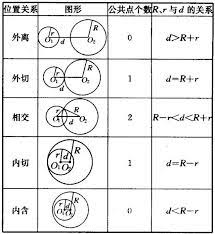# 1376: Ifrit的家庭作业

### 题目描述

`Ifrit`今天学到了圆的概念，家庭作业中自然都是相关的内容。

```c++

# include

using namespace std;

struct Coordinate { double x; double y; };

struct Circle { Coordinate center; double radius; };

const int MAX_NUM = 10000; int circleNumber = 0; // 目前存储的圆的数量 Circle circles[MAX_NUM];

``````circleNumber++;
``````

}

void modifyCircle(int index, Coordinate center, double radius) { // todo

}

void printCircle(Circle circle) { // todo

}

bool intersect(Circle circle1, Circle circle2) { // todo

}

void traverse() { // 使用指针遍历数组 for (Circle ptr = circles; ptr != circles + circleNumber; ptr++) { printCircle(ptr); } }

int main() { int op, n; cin >> n; while (n--) { cin >> op; if (op == 1) { double x, y, r; cin >> x >> y >> r; Coordinate center; center.x = x; center.y = y; addCircle(center, r); } else if (op == 2) { int index; double x, y, r; cin >> index >> x >> y >> r; Coordinate center; center.x = x; center.y = y; modifyCircle(index, center, r); } else if (op == 3) { int index; cin >> index; printCircle(circles[index]); } else if (op == 4) { int index1, index2; cin >> index1 >> index2; if (intersect(circles[index1], circles[index2]))cout << "Yes" << endl; else cout << "No" << endl; } else if (op == 5) { traverse(); } } }

```

### 输入格式

##### op == 2 : 更改某个圆的属性

`0-based`并保证`index`不会越界。

##### op == 3 : 输出某个圆的信息

`0-based`并保证`index`不会越界。

##### op == 4 : 判断某两个圆是否相交

`0-based`并保证`index1``index2`不会越界。

### 样例输入

```6 1 1 1 2 1 0 0 2 3 1 2 1 -1 -1 3 4 0 1 5```

### 样例输出

```0.000 0.000 2.000 Yes 1.000 1.000 2.000 -1.000 -1.000 3.000```

### 数据范围## Oops! 本题目还没有解答！

OJ翻了一新，但本解答集还大多用的是2017-2019级，甚至更早的同学们贡献的答案。